# Common Core: High School - Geometry : Modeling with Geometry

## Example Questions

← Previous 1 3 4

### Example Question #1 : Use Geometric Shapes To Describe Objects: Ccss.Math.Content.Hsg Mg.A.1

Identify all of the different shapes that make up the following figure of a two-dimensional boat.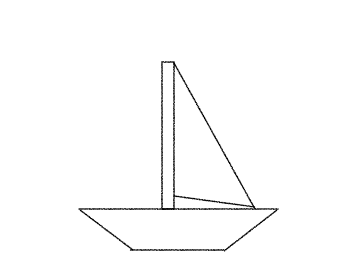Triangle, Rectangle

Square, Trapezoid, Triangle

Polygon, Rectangle

Trapezoid, Equilateral Triangle

Trapezoid, Triangle, Rectangle

Trapezoid, Triangle, Rectangle

Explanation:

In the world around us, all figures can be looked at in geometric shapes.

Looking at the two-dimensional boat,the boat can be broken down into three geometric shapes. The bottom of the boat is a trapezoid, the pole in the center of the boat is a rectangle and the sail is a triangle.

### Example Question #1 : Modeling With Geometry

Identify all of the different shapes that make up the following figure of a two-dimensional wagon.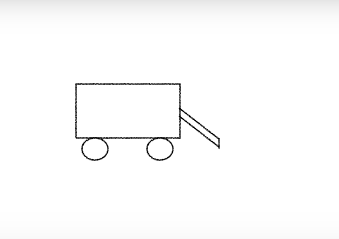Circle, Square, Parallelogram

Parallelogram, Rectangle

None of the answers

Rectangle, Circle

Explanation:

In the world around us, all figures can be looked at in geometric shapes.

Looking at the following  two-dimensional wagon,the figure can be broken down into three different shapes. The body of the wagon is a rectangle, the wheels are seen geometrically as circles and the handle is a quadrilateral.

Recall that to be a square, the quadrilateral has to have four equal side lengths.

A parallelogram must have opposite parallel sides, this particular figure doesn't give enough information to determine whether the handle is a parallelogram.

A quadrilateral is a more correct statement as it describes a figure with four sides.

Therefore, the correct answer is, "Rectangle, Circle, Quadrilateral".

### Example Question #3 : Use Geometric Shapes To Describe Objects: Ccss.Math.Content.Hsg Mg.A.1

Identify all of the different shapes that make up the following figure of a two-dimensional flagpole.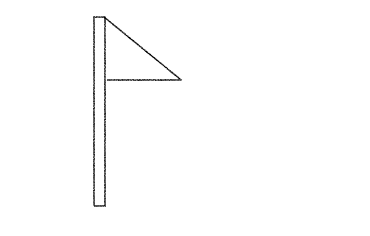Square, Trapezoid

Square, Triangle

Rectangle, Trapezoid

None of the other answers

Rectangle, Triangle

Rectangle, Triangle

Explanation:

In the world around us, all figures can be looked at in geometric shapes.

Looking at the two-dimensional flagpole,the flagpole can be broken down into two geometric shapes. The pole portion of the flagpole can be described as a rectangle and the flag is in the shape of a triangle.

### Example Question #4 : Use Geometric Shapes To Describe Objects: Ccss.Math.Content.Hsg Mg.A.1

Identify all of the different shapes that make up the following figure of a two-dimensional stop light.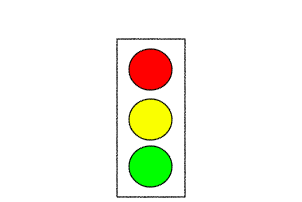Rectangle, Trapezoid

Square, Circle

None of the other answers

Rectangle, Circle

Rectangle, Triangle

Rectangle, Circle

Explanation:

In the world around us, all figures can be looked at in geometric shapes.

Looking at the two-dimensional stop light,the figure can be broken down into two geometric shapes. The outside portion of the stop light is a rectangle and the lights are in the form of circles.

### Example Question #5 : Use Geometric Shapes To Describe Objects: Ccss.Math.Content.Hsg Mg.A.1

Identify all of the different shapes that make up the following figure of a two-dimensional non traditional stop sign.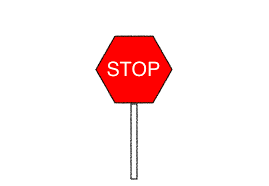Rectangle, Square

Octagon, Rectangle

None of the other answers

Hexagon, Rectangle

Hexagon, Octagon

Hexagon, Rectangle

Explanation:

In the world around us, all figures can be looked at in geometric shapes.

Looking at the two-dimensional non traditional stop sign,the figure can be broken down into two geometric shapes. It is important to point out that this stop sign is a non traditional one. Normal stop signs are octagons which have eight sides. This particular stop side only has six sides and is therefore a hexagon. Therefore, this non traditional stop sign can be broken down into a hexagon and a rectangle.

### Example Question #1 : Use Geometric Shapes To Describe Objects: Ccss.Math.Content.Hsg Mg.A.1

Objects in the real world can be described in geometric shapes. What geometric shape describes a water silo?

Cylinder

Sphere

None of the other answers

Triangle Prism

Rectangle Prism

Cylinder

Explanation:

In the three-dimensional world a water silo has a bottom and top in the shape of a circle. The three-dimensional connection between the top and the bottom creates a cylinder. Therefore, the geometric shape that best describes the silo is a cylinder.

### Example Question #1 : Use Geometric Shapes To Describe Objects: Ccss.Math.Content.Hsg Mg.A.1

Objects in the real world can be described in geometric shapes. What geometric shape describes a basketball?

Circular Prism

Rectangle

Sphere

Circle

Cylinder

Sphere

Explanation:

A basketball is a three-dimensional object that when cut down the middle has a circular base. The geometric shape that describes a basketball is known as a sphere.

### Example Question #1 : Use Geometric Shapes To Describe Objects: Ccss.Math.Content.Hsg Mg.A.1

Sally orders a pair of shoes from an online store. The box that the shoes arrived in can be described as what geometric shape?

None of the others.

Triangular Prism

Cylinder

Sphere

Rectangular Prism

Rectangular Prism

Explanation:

A box in three-dimensional space can be describes as either a cube or a rectangular prism depending on the side lengths. Since rectangular prism is the only option available, this is the best option. Most shoe boxes in general are rectangular prisms since shoes are longer than they are wide.

### Example Question #6 : Use Geometric Shapes To Describe Objects: Ccss.Math.Content.Hsg Mg.A.1

Identify all of the different shapes that make up the following figure of a two-dimensional snowman.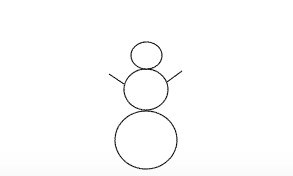Oval, Triangle

Circle

None of the other answers.

Circle, Line

Line

Circle, Line

Explanation:

In the world around us, all figures can be looked at in geometric shapes.

Looking at the two-dimensional snowman,the figure can be broken down into one main geometric shape, a circle. The base, torso, and head of the snowman are all circles and the arms are lines. Therefore, the geometric shapes of the two-dimensional snowman are circles and lines.

### Example Question #7 : Use Geometric Shapes To Describe Objects: Ccss.Math.Content.Hsg Mg.A.1

Identify all of the different shapes that make up the following figure of a three-dimensional open box.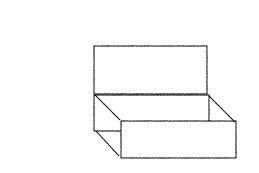None of the other answers

Cube

Rectangular Prism, Rectangle

Rectangular Prism, Cube

Cube, Rectangle

Rectangular Prism, Rectangle

Explanation:

In the world around us, all figures can be looked at in geometric shapes.

Looking at the three-dimensional open box,two figure can be broken down into two main shapes, one which is a three-dimensional shape and the other is a two-dimensional shape. The box itself can be described as a rectangular prism. The lid of the box, which is up and thus making the box open is a rectangle.

← Previous 1 3 4

### All Common Core: High School - Geometry Resources